Welcome to the Algebra II Zone

(Algebra II, Blocks 2 and 3)

Are you nervous when you see NCWEE? concerned when you see CIRC? perturbed when you see PBC? Visit Mr. Hansen’s fabled abbreviations page to make sense of those cryptic markings you see on your papers.

 Schedule at a Glance (see archives for older entries) Written assignments should follow the W 1/20/16 D Classes resume. Th 1/21/16 E HW due: 1. If you did not have Mr. Hansen last semester, log in to the e-mail service you check most frequently, and send Mr. Hansen an e-mail message with the following subject line: __Hello from [your name] Be sure that you put 2 underscore characters before the word “Hello,” exactly as shown. Be sure to include your name, since it is not always possible to determine a person’s name from an e-mail address alone. In the body of your message, please write something that Mr. Hansen should know about you. 2. Write §5.1 (p. 434) #1-25 odd, 27-32 all, 33, 35. Try to follow the HW guidelines (see link at top of schedule). New students will have their HW reviewed and critiqued; all others will be expected to follow the same guidelines from last fall. F 1/22/16 F Snow day. The assignment originally due today (Block 2) has been postponed until Monday. If Monday also turns out to be a snow day, you will need to check this page for additional assignments that will be due when school resumes. M 1/25/16 G Snow day. However, the following assignment is still due today: 1. Read §§5.1 and 5.2 (pp. 422-447). Reading notes are required, as always. 2. Continue to monitor www.modd.net for additional assignments if the snow break continues beyond the weekend. T 1/26/16 A Snow day. However, the following assignment is still due today: 1. Make sure you have completed all the assignments above. The e-mail requirement (see 1/21 calendar entry above) has not yet been met by Malcolm S. 2. Write §5.1 (p. 435) #49-59 odd, 66-74 all, 81. Yes, word problems with exponential functions are a little trickier than the word problems you did last semester involving direct variation, joint variation, and inverse variation. No, exponential models are not much trickier, and no, you do not have to have a special class period taught by Mr. Hansen in order to figure out how to work with them. You may copy the solution below for #49 if you wish (no penalty): 49. Given: Pop. doubles every 88 years.       Let x = # of years elapsed since 2002 (0 means 2002, 1 means 2003, etc.).       Let y = predicted pop. (millions)       Model: y = f (x) = k · 2x/88       [Reasonableness check: f (0) = k · 20/88 = k · 20 = k, and f (88) = k · 288/88 = k · 21 = 2k. Both OK.]       By insp., k = 7.1, since we want f (0) = 7.1 and f (88) = 14.2.       Want pop. in 2050, i.e., f (48).       f (48) = k · 248/88                 = 7.1 · 248/88                 =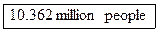[Note: Clearly, rounding to 1 decimal place to get 10.4 million is appropriate. However, this is not a science class. To train you for possible AP exams in calculus or statistics that you may take someday, we will always give answers correct to at least 3 decimal places after the decimal point.] Do you see how doing a problem like this is almost exactly the same technique that you used last semester when solving word problems involving direct, joint, and inverse variation? The only real change is that when writing down a model, you will write down something like y = k · ax instead of y = kx or y = k/x. W 1/27/16 B HW due: 1. Send e-mail to Mr. Hansen for any of the problems due Tuesday that you were unable to solve. Use the following subject line: __Section 5.1 Q&A from [your name] Note that the leading two underscore characters in the subject line are required. Mr. Hansen receives hundreds of e-mails in a typical day and will never find student e-mails amidst the chaff unless you use a uniform convention to make your e-mails stand out. Also, if your e-mail gets routed to the spam bin by mistake, having a uniform subject line makes it much easier to find among the spam. Putting your name in the subject line makes it much easier for Mr. Hansen to track threads from multiple students. Because you are all 15 or 16 years old and can follow instructions if you put your mind to it, any e-mails whose subject lines do not conform to the formatting requirement shown above may be ignored. If you do not receive a response within a reasonable amount of time and have followed the instructions for formatting your subject line, you may assume that your e-mail has been incorrectly identified as spam, and you may leave a message on Mr. Hansen’s voicemail. (See contact information on modd.net home page.) 2. Write §5.2 (pp. 447-448) #1-63 odd, 65-70 all. The first two in the first two groups are done for you as examples, and you may copy them. Note that you cannot simply write the answer, since the setup is part of what you must write in each case. The symbol “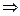” means “implies” and is a substitute for starting a fresh line. 1. log5 125 = 3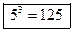3. log81 3 =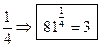21. 83 = 512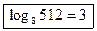23. 0.00001 = 10–5[no need to indicate base since log means log10] Th 1/28/16 C No class. F 1/29/16 D HW due: 1. Read §5.3 (pp. 451-457). 2. Write §5.2 (pp. 448-450) #72-80 eoe (use calculator to check after you have made each sketch by hand, with at least 2 points labeled in each case), 83, 89, 92 (OK to use 91 as a hint), 99, 102-108 all. M 2/1/16 E HW due: 1. Read §5.4 (pp. 460-467). 2. Write §5.3 (p. 458) #3-30 mo3, 45-54 all (OK to use calculator for #45-54, but please do not use the logBASE function except as a cross-check). Note: Show work for those that cannot be solved by inspection. For example, #17 was not assigned, but here is how you would do it: 17.T 2/2/16 F HW due (Block 2 only) 1. Write §5.3 (pp. 458-459) # 55, 56, 59-66 all, 68, 70. Hint: #68 is rather tricky. However, if you can’t get #68 to work out, you can still use it as a corollary to solve #70 in a snap. W 2/3/16 G HW due (Block 3 only): See yesterday’s calendar entry. Th 2/4/16 A HW due: 1. Without using WolframAlpha.com, prove that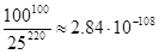, where the approximation is correct to 3 significant figures. You may use your calculator and your knowledge of logarithms. 2. Write §5.4 (pp. 467-468) #5-75 mo5. 3. Use the Dep-Scribble-SwykEA process to solve problems #85-88 all (all on p. 468). F 2/5/16 B HW due: 1. Finish the assignment that was due yesterday. Note: You cannot use a placeholder for credit today on problems such as #88, which is easily solved using the 2nd CALC Intersect feature of your calculator. 2. Write the following additional problems in §5.4 (pp. 469): #91-98 all. M 2/8/16 C No class. T 2/9/16 D HW due: 1. Read §5.5 (pp. 470-476). At the beginning of your reading, make the following changes to your textbook in pencil (or in pen, if you prefer): (a) For the first 4 standard models on p. 470, make a note in your textbook that c > 0. These models are virtually always used for positive quantities (population, density, mass, etc.), and therefore c > 0 should be stated as a ground rule. (b) For the second standard model on p. 470, exponential decay, delete the minus sign and change the note to say that k < 0. In other words, we will make our lives simpler if we consider only one standard model and let the sign of k determine whether we are modeling growth or decay. The standard model for all exponential word problems is y = cekx, and the only thing we have to remember is that if k > 0, we have growth, and if k < 0, we have decay. (c) For the fifth standard model on p. 470, cross out the function f (x) = a + c log x. We never need this, since the common log is simply a constant times the natural log (ln) and can therefore be absorbed in the constant. Optional extra credit (1 point): Explain why the common log of any positive number is always simply a constant times the natural log of that number. (d) For the fifth standard model on p. 470, change the c in f (x) = a + c ln x to b. In other words, the function should be f (x) = a + b ln x. (The reason will become clear later on.) (e) On p. 474, near the top of the page, cross out the solution to part (c). This is not the correct way to find the predicted number of boys who weigh between 94.5 and 95.5 pounds. The correct method is taught in AP Statistics or in any college statistics class, and that will be at least 2 years in the future for you. As a result, we will use Gaussian models only for sketching the overall shape of a distribution, not for doing computations of the type shown in part (c). 2. We have looked at exponential functions of the form y = Bx, where B denotes a base that must be positive but not equal to 1. Common values for B are 10, 2, and e. If 0 < B < 1, we have exponential decay, whereas if B > 1, we have exponential growth. In §5.5, we will enlarge our definition of exponential functions to make them more flexible to fit more real-world problems. Our new definition, found on p. 470, is to rewrite all exponential functions in terms of e. As you can see on your marked-up version of p. 470, all exponential growth problems can be modeled by a function of type y = cekx, where c and k are constants chosen to match the requirements of the problem, with c > 0 and k > 0. Similarly, all exponential decay problems can be modeled by a function of type y = cekx, where c and k are suitable constants, with c > 0 and k < 0. (a) Prove that for any positive real number q and for any real number r, qr = er ln q. Note: This is a quick proof, but if you can’t get it, you are NOT PERMITTED to stop. Instead, you should accept the fact that qr = er ln q as a given and move on, since you will need this fact for parts (b) and (c). (b) Consider the exponential growth equation y = 1.08x, which models the value of a \$1 investment at an 8% compound annual return. Rewrite this equation in the standard form shown on p. 470 with k > 0. (c) Show that the exponential decay equation y = 100 · 0.85x, which models the percentage of unsold and unreplaced inventory that remains in a store with a 15% annual “shrinkage” problem, results in 85% remaining after year 1, 72.25% remaining after year 2, and about 61.4% remaining after year 3. (“Shrinkage” refers to theft, breakage, rotting, and all other losses except for legitimate sales.) (d) Consider the exponential decay equation given in part (c). Rewrite this equation in the standard form shown in your marked-up version of p. 470, with k < 0. (e) Let us accept as a given that all exponential growth or decay problems can be rewritten in the standard form y = cekx, as listed on your marked-up version of p. 470. Prove that the inverse function is always of the form y = a + b ln x, where a and b are constants. Optional extra credit (1 point): Prove that in part (d), the inverse function y = a + b ln x always has b < 0 if k < 0, whereas b > 0 if k > 0. 4. Write #7 and #13 on p. 477. 5. Comment briefly on #13. (Hint: It helps if you paid attention to Mr. Hansen’s chapel talk on Dec. 7, or if you have seen The Big Short, or if you paid attention to anything happening during the year 2007.) W 2/10/16 E HW due: 1. Read the following solution by Mr. Hansen of #25 on p. 478. Then, apply the same ideas to solve #26, #27, and #28. 25. Let TS = 72 = surrounding temp. (in °F.)       Let T0 = 325 = initial temp. of pie (in °F.), assuming the pie was in the oven for a long time       Apply Newton’s model: T(t) = TS + (T0 – TS)e–kt       Plug in to get T(t) = 72 + 253e–kt       SWYK: We know T(10) = 72 + 253e–k(10) = 200       Algebra: 253e–k(10) = 200 – 72                      253e–10k = 128                      e–10k =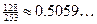[Note: Three dots indicate additional accuracy stored in calc.]                      ln e–10k = ln(0.5059...)                      –10k = ln(0.5059...)                      –10k = –0.681...                      k = 0.0681...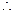Particular model is T(t) = 72 + 253e–0.0681...t       Plug in t = 30 to get T(30) = 104.763... or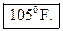to nearest degree. 2. Write the following chapter review exercises on pp. 484-487: #9, 11, 13-16 all, 25, 26, 29-40 all, 45-56 all, 68-94 even, 103, 105. Note: There are too many to do all in one night. This assignment may be continued until Thursday if necessary. Keep a time log. Th 2/11/16 F HW due (Block 2 only): Finish the problems assigned for yesterday. F 2/12/16 No school (faculty professional day). M 2/15/16 No school (holiday). T 2/16/16 G HW due (Block 3 only): Finish the problems assigned for last Wednesday. W 2/17/16 A HW due: 1. Finish any remaining problems from the review set assigned for last Wednesday, a week ago. Correct answers are expected for all problems, since you have had more than a week to work on these. 2. Skim §6.1 and §6.2 (pp. 492-520). You are not expected to read every word, since virtually all of this material should be familiar to you from previous classes. The only new terms are secant (the reciprocal of the cosine function), cosecant (the reciprocal of the sine function), and cotangent (the reciprocal of the tangent function). You are expected to be familiar with the definitions and abbreviations of the 6 functions listed in the light brown box on p. 511. Reading notes are required, as always. Note: Form III students are permitted to show extremely brief reading notes because of the class field trip to Ford’s Theatre. We would have had the test today if it had not been for that field trip. Th 2/18/16 B Test (100 points) on Chapter 5. F 2/18/16 C No class. M 2/22/16 D HW due: 1. Prepare for oral quizzing: §6.1 (p. 504) #1-15 all, §6.2 (p. 520) #13-24 all. 2. Write §6.1 (pp. 504-506) #17-69 eoo (“every other odd,” i.e., 17, 21, 25, etc.) 3. Write §6.2 (pp. 521-523) #39-50 all, 55-62 all, 91-94 all. There will be a 7:00 a.m. retest later this week for students who failed last Thursday’s test. The date will be posted soon. T 2/23/16 E HW due: 1. Read §6.3 (pp. 524-531). 2. Prepare for a possible open-notes quiz on significant digits. (For example, 0.04 has 1 significant digit, whereas 38 has 2 and 38.0 has 3.) 3. Prepare for a possible closed-notes quiz on basic calculator functionality (understanding scientific notation, STOing values into variables, evaluating functions accurately, plotting in a suitable window). W 2/24/16 F Retest on Chapter 5: Required for all students whose score written on the first page on last week’s test was below 65. The retest will begin in MH-102 at 7:30 a.m. sharp. (This is a change, since the faculty meeting starts at 8:30 instead of the usual 8:00.) Please arrive 5 minutes early so that we can start promptly at 7:30. Note 1: After a review of all scores for both classes (mean of 60 in Block 2, 68 in Block 3), it seemed prudent to add a 5-point general curve to everyone’s score. Thus you should not come at 7:30 if the score written on the first page of your test was 65 or higher. After the adjustment, your score will be 5 points higher than the score written on the first page of your test. Note 2: The retest is not intended for everyone who wants to boost his score, since the classroom is not big enough to hold everyone who wants to boost his score. The purpose of the retest is to give people who had major difficulties on the original test a chance to move into the passing zone, period. Optional Point-Boosting Opportunity for all other students will begin at 8:10 a.m. sharp. Please wait quietly in the hallway until 8:10. At 8:10, the door will fly open, and you will have a chance to do one or two problems or proofs. If you do well, points will be added to your test score. If you do poorly, your test score will stay as it is. If you cannot attend either event, for illness or appointment or other reason, please e-mail Mr. Hansen immediately (with double underscore __ at the beginning of the subject line) to make alternate arrangements. An alternate “point-boosting opportunity” will be held in MH-102 during flex time, 11:25-11:45, by invitation only. Most people who want to boost their score need to show up at 8:10. Practice tests (blank copies) are available here: Version A (Block 2)   Version B (Block 3) Solution keys are available here: Version A (Block 2)  Version B (Block 3) HW due (Block 2 only): Write §6.3 #1-45 eoo. Th 2/25/16 G HW due (Block 3 only): Write §6.3 #1-45 eoo. F 2/26/16 A HW due: 1. Read §6.4. 2. Be sure that you have completed all the previously assigned problems in §6.3. (This assignment has been corrected in the previous calendar entries to #1-45 eoo, not #1-47 eoo, since #47 does not come up in the sequence.) 3. Prepare for oral presentation: §6.4 (p. 549) #1-17 all. A special thank-you to Will P., Will A., and Matthew D. for teaching the first 15 minutes of yesterday’s Block 3 class! M 2/29/16 B Before school, at 7:10 a.m. sharp: A special “Re-Retest” will be held, by invitation only, for people who continued to struggle on last Wednesday’s retest. Send e-mail if you are unsure as to whether or not you qualify. HW due: 1. Write §6.4 (pp. 549-551) #73-95 odd, 103-116 all. 2. (Optional, but well worth it.) Watch this video about a scale model of our solar system. Note: At the same scale used in the video, the next closest star, other than our sun, would be more than 10,000 miles away. T 3/1/16 C No class. W 3/2/16 D HW due: 1. Read §6.5 (pp. 552-564). 2. Write §6.5 (p. 564) #1-10 all. A sketch for each question is required. Th 3/3/16 E HW due: 1. Write §6.5 (pp. 564-565) #11-24 all, including the ones that we already did in class. 2. Write §6.5 (pp. 565-567) #47-69 odd, 77, 78, 91, 104. F 3/4/16 F HW due (Block 2 only): 1. Read §6.6 and §6.7. Reading notes are required, as always. Most, but not all, of this reading is review of the skills we already learned when building the Trig Tracker card. 2. Be prepared for a quiz on trig facts. You will be permitted to use your Trig Tracker card if you wish, but you must work toward performing the skills without notes in the future. Sample question: What angle(s) having nonnegative radian measure less than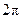can satisfy the equation sin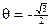? Give answer(s) in degrees and radians. Answer: Using our knowledge that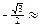–0.866, we have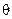= 240° or= 300° from our Trig Tracker card. In radian measure, those angles are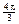or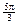, respectively. Note: If the problem had not specified the domain restriction for, we would have to consider adding or subtracting all possible whole-number multiples of 360° from those answers, giving the following solution set for: {240°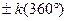}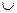{300°}. In radians, the solution set would be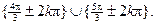In both solution sets, the letter k is used to indicate all possible choices of whole numbers. (Recall that the set of whole numbers is defined to be the set of nonnegative integers, namely {0, 1, 2, 3, . . . }.) M 3/7/16 G HW due (Block 3 only): See last Friday’s entry for the other class. T 3/8/16 A HW due: 1. Write the following review exercises. If you do not finish every last one, leave placeholders and a time log. We will go over as many as possible in class. You should be sure to do all the review problems before taking the test on Wednesday. p. 652 #5, 9, 13, 16, 18, 19, 25 p. 653 #55, 63, 97-110 all 2. Prepare orally: p. 652 #35-46 all p. 653 #67-72 all, 81-96 all 3. Write the following extra problems: E1. Solve the right triangle having one leg of length 5 m and a hypotenuse of length 7 m. Give angles in both degrees (nearest thousandth of a degree) and radians (nearest thousandth). E2. A car’s camshaft is rotating at 3200 rpm. Compute the angular velocity in both radians per second and degrees per second. E3 (Block 3 only, since the fire drill on 3/7 took part of our class period.) Sketch the tangent function, y = tan x, where x is in radians. You may use your calculator to help you if necessary. Show that the domain on which the curve is one-to-one is almost exactly the same as the domain on which y = sin x is one-to-one. Use this fact to sketch the graph of y = arctan x. (Note: The word “arctan” is simply another name for the inverse tangent function. We say y = arctan x if y is the unique angle strictly between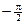and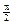radians whose tangent equals x.) In class: Review. W 3/9/16 B Test (100 pts.) on §§6.1 through 6.7. Angular velocity is included, but only the easier types of problems (see, for example #E2 in yesterday’s review set). You will be permitted to use your calculator and Trig Tracker card for most of the test, but a few problems at the beginning will be “no calc, no notes.” Th 3/10/16 C No class. F 3/11/16 D HW due: Read §6.8 (pp. 595-615 and the summary on p. 618 only; skip addition of ordinates on pp. 615-617). Much of this can be skimmed, since we have already discussed it. However, you will definitely want to learn the tan box on p. 603, the four bullets at the bottom of p. 607, and the tan box on p. 608. Reading notes are required, as always. M 3/14/16 E Pi Day. (Remember,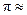3.1416. Get it?) In honor of Pi Day and the first warm weekend of the year, there is no additional HW due today. Hooray! T 3/15/16 F HW due (Block 2 only): Not posted in time because of a technical glitch. W 3/16/16 G HW due (Block 3 only): 1. Read §6.9. You should read pp. 624-632 (middle) 638). Correct the typo in the first sentence of p. 626: “increases” should be “decreases.” Reading notes are required, as always, but you may skim the material from the middle of p. 632 onward, since it is relatively less important than the earlier material. 2. Write #41-48 all on pp. 619-620. Do all 8, including the ones that were done in class. There is no need to show a sketch, but you do need to explain your reasoning. The first one is done for you as an example: 41. Like sine, but flipped. Period =, or 2 times the usual freq. Answer: y = –sin 2x. 3. Write #97, 98, and 109 on pp. 621-622. Th 3/17/16 A HW due: Block 2: Do everything that was assigned for Block 3 (see yesterday’s calendar entry), plus the following problems from §6.9 (pp. 639-641): #1-8 all, 56-68 all. Block 3: Finish yesterday’s assignment if you have not already done so. Then, write the following problems from §6.9 (pp. 639-641): #1-8 all, 56-68 all. Bonus opportunity for both sections (2 points): Derive the equation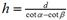, the parameterized solution that solves all problems of the type shown in the colorful mountain sketch on p. 641. The only skills required are algebra and the ability to work with tangent and cotangent functions. F 3/18/16 B HW due: 1. Read pp. 663-665, the box in the middle of p. 686, the box at the bottom of p. 688, the shaded table on p. 695, and pp. 720-729 (only to the middle of p. 729). That’s all we’ll be looking at in Chapter 7. 2. Write p. 760 #15-25 odd, 29-31 all, 107-111 odd, 119-130 all. Then, close the book on Chapter 7. You may use your Trig Tracker card to help you. Spring break M 4/4/16 C No class. T 4/5/16 D Classes resume. Come to class with your learning attitude primed for extreme learning excitement in Q4! W 4/6/16 E HW due: 1. Read §8.1. Reading notes are required, as always. 2. Write §8.1 (p. 781) #1-6 all, 13. Note: You do not need to understand the content of §8.1 (which will be taught today in class) in order to do #13. All you need is an ability to sketch (a triangle with auxiliary lines) and a recollection of your basic 30°-60°-90° facts from geometry. 3. Optional bonus (2 pts.): Use trigonometry to solve #7. This is harder than #13, but again, it does not require any of the advanced techniques taught in §8.1. 4 (required for everyone). In class yesterday, we saw that log5 x =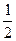log25 x, that log9 x =log3 x, and that log27 x =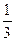log3 x. From these and other observations, we came up with a class conjecture: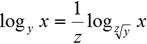Your assignment: (a) Be absolutely clear about what the acceptable values (the “givens”) are for x, y, and z. (b) Prove or disprove the class conjecture. I will not tell you whether it is true or false, or whether it is true only for some values and false for others. Note: Working with classmates is acceptable. For this problem (and this problem only), copying a line or two from someone else’s work is permitted. However, each student must produce a full writeup written from his own pencil, and the names of collaborators must be acknowledged. Th 4/7/16 F HW due (Block 2 only): 1. Read §8.2. Reading notes are required, as always. 2. Write §8.1 (pp. 781-784) #11, 21, 27, 51, 52. 3. In the Tower of Pisa example (p. 780), the fifth substep of Step 1 says, “Solve for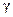using the inverse sine function.” However, there are actually two angles between 0 and 180 degrees that have a sine of 0.643619463. (a) Find the other possible angle. (b) Why did the book completely gloss over the angle you found (or should have found) in part (a)? Write a complete sentence or two, in your own words. F 4/8/16 G HW due (Block 3 only): 1. Read §8.2. Reading notes are required, as always. 2. Write §8.1 (pp. 781-784) #11, 21, 27, 51, 52. 3. In the Tower of Pisa example (p. 780), the fifth substep of Step 1 says, “Solve forusing the inverse sine function.” However, there are actually two angles between 0 and 180 degrees that have a sine of 0.643619463. (a) Find the other possible angle. (b) Why did the book completely gloss over the angle you found (or should have found) in part (a)? Write a complete sentence or two, in your own words. M 4/11/16 A HW due: 1. Read §8.3 (pp. 796-800). Reading notes are required, as always. 2. Write §8.1 (p. 782) #41, using the Law of Sines. A diagram is also required. 3. Write §8.2 (pp. 792-795) #17ab (see below), 25, 47, 61, 62. Note: For #17, solve the problem two ways: (a) by using the Law of Cosines (b) by using a faster method (explain your reasoning). T 4/12/16 X Diversity Forum (no class). W 4/13/16 B HW due: 1. Read §8.4 (pp. 804-814). Reading notes are required, as always. 2. On the middle on p. 804, change the word “former” to “latter.” We will use the notation ||u|| for the magnitude of vector u, despite what your book says. 3. On p. 804, change the notation for the vector magnitude from |u| to ||u|| for both occurrences on that page. 4. Near the top of p. 805, change |u| and |v| to ||u|| and ||v||. 5. On p. 806, change all 7 occurrences of |u| to ||u|| on that page. Note: Hereafter, whenever we see the notation |u| being used to refer to the magnitude (a.k.a. norm) of a vector, we will think of ||u||, but we won’t take the time to make the change in our textbook. 6. You may wonder how we will indicate boldface type in our written work when we refer to vectors by their letter names. (No, we won’t bear down harder to make boldface pencil letters!) The standard used by most mathematicians and engineers is to put a little arrow over the letter name of the vector, like this: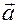If you prefer, you can make a “shortcut arrow,” like this: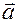That gives us a way of distinguishing between the variable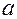, which is a number, and the vector, which is a vector with two components, for example, <3, –4>. Your exercise is to prove that for any two vectors,and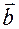, we have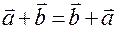. Be sure to state the “givens” and the “prove” statement before you begin your proof. Hint: Without loss of generality, put as the second step in your proof thatis represented by the coordinates andby the coordinates . 7. Write the coordinates of the zero vector, namely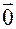. Remember to put angle brackets, not regular parentheses, around your answer. 8. Your book deals only with vectors in 2 dimensions, but there is nothing to stop us from having 3, 4, 5, or even 100 or more dimensions. (There are even infinite-dimensional vector spaces!) Although we will usually stick to 2 dimensions to keep things manageable, write the formula for ||u|| if u is the 3-dimensional vector <3,–4,12>. Show work and simplify your answer. Hint: Think back to geometry class. The norm of a 3-dimensional vector is the same as the diagonal length for a 3-dimensional rectangular solid. Th 4/14/16 C No class. F 4/15/16 D HW due: 1. Write §8.3 (pp. 800-801) #1, 7, 13, 19, 25, 40, 43. 2. Write §8.4 (pp. 814-815) #1-5 odd, 17-23 odd, 35-43 odd, 51-55 odd. 3. Write an answer to the Monty Hall problem. Be prepared to justify your reasoning. 4. Write answer to both versions (A and B) of the Chest of Drawers problems. Be prepared to justify your reasoning. M 4/18/16 E HW due: 1. Download and print out this worksheet. Punching the edges with a 3-hole punch is recommended (so that you can keep the worksheet in your 3-ring binder), but there will be no point deduction if you skip that step. If you don’t have a printer, it is acceptable to write the setups and answers on a regular set of HW sheets. 2. Despite what the instructions say, do not write your name where indicated on page 1. Instead, write your name in the upper right corner of page 1 (as you always do), along with the word “due” and the due date:                                        due 20160418 3. If you get stuck on the Venn diagrams on page 1 or 3, read the material below. If you still can’t figure out how to fill in the Venn diagrams, phone a classmate or send e-mail to Mr. Hansen. Be sure to include a double underscore (looks like this: __ ) at the beginning of your subject line so that your message is less likely to be rejected as spam. 4. On the final page, there is a typo in question #4(b). Add a vertical bar (the “given” bar) as shown here: (b) P(2nd card is a jack | 1st card is a jack) 5. For question #4(c), note that we already solved the problem in class, both by a priori analysis and with a computer simulation. You should certainly be able to answer this one correctly! 6. In question #4(e), if you are not a card player, you may not know what the phrase “two of a kind” means. The question is asking for the probability that you obtain two cards of the same value (for example, ace of clubs and ace of spades, or 10 of diamonds and 10 of spades). One way to analyze this problem is to imagine that the first card has been chosen, whatever it happens to be, and you have to draw as your second card one of the other 3 cards of that same value from the remaining cards. Better yet, run a simulation! 7. You have probably seen Venn diagrams in previous classes, but if not, here is a filled-in Venn diagram that you can use to get started on your assignment: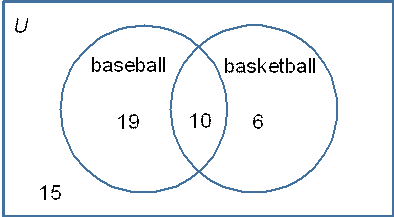The letter U denotes the universe of all 50 students. Circles denote the subsets of students who play baseball and basketball, respectively. Note that 29 (a total of 19+10) students play baseball, while 16 (a total of 6+10) play basketball, as required. The diagram also shows 10 students in the intersection region, namely (baseball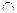basketball). Finally, since the problem told us that there are 50 students, we have to stick the leftover 15 students in a portion of the diagram that is outside both the baseball and the basketball regions. In other words, there must be 15 students who play neither baseball nor basketball. 8. (Optional.) The challenge problem is optional but is strongly recommended for those who have spoken to Mr. Hansen about possibly jumping to the honors track next year. For the challenge problem, assume that the value of an ace is 11. The 10, jack, queen, and king all have a value of 10. T 4/19/16 F HW due (Block 2 only): 1. Read §12.6 (pp. 1167-1174), especially the calculator “Technology Tips” in the margins of pp. 1172-1173. Reading notes are required, as always. Note: There is a typo in the Technology Tip in the margin of p. 1171. The first “6” under the section marked “Graphing calculators:” should be 13, not 6. 2. Write answers to the following questions. Use your calculator. Your “work” should consist of your setup, using standard notation. The first one is done for you as an example. For problems involving combinations, please show both the “nCr” format and the “parentheses” format for combinations, since as shown at the top of p. 1172, the notations are equivalent. You should be equally comfortable with both formats, though, since both are in common use. (a) How many 13-card bridge hands are possible? Solution:(b) How many 5-card hands are possible in draw poker, with no jokers? (c) How many Virginia license plates of the form XXX #### are possible? (Here, “X” denotes a letter, and “#” denotes a digit, 0-9.) (d) How many 3-student student council delegations (with all ranks considered equal) can be chosen from a Form IV class of 79 students? (e) How many 5-student student council delegations (with distinguishable ranks of Head Prefect, President, Vice President, Prefect 4, and Prefect 5) can be chosen from a Form V class of 80 students? (f) How many 5-digit student IDs are possible if the first digit must be a 2 or a 3, and if the remaining digits are allowed to be any digit, 0-9? (g) How many Internet addresses are possible if an address consists of 4 integers, separated by dots? (For example, 172.8.15.255 is an Internet address.) Each of the 4 integers must be between 0 and 255, inclusive. (h) How many different ways can a hamburger be assembled if each burger may include (or not include) any or all of the following toppings: ketchup, mustard, lettuce, tomato, grilled onion, raw onion, mayonnaise, grilled mushrooms, cheese, jalapenos? Note: All burgers must include a bun and a beef patty. Those are the only required elements. All of the toppings are optional. The order in which toppings are applied does not matter. For example, lettuce with tomato and then ketchup applied over the top of the tomato is considered equivalent to ketchup directly on the patty and lettuce and tomato piled on top. (i) If the only possible Republican presidential candidates for 2016 are Trump, Cruz, and Kasich, and if the only possible Democratic presidential candidates for 2016 are Clinton and Sanders, how many different 2-way contests are possible? W 4/20/16 G HW due (Block 3 only): See yesterday’s calendar entry. Th 4/21/16 A HW due: 1. Write §12.6 (pp. 1174-1175) #19, 27, 41-46 all. 2. Write §12.7 (pp. 1184-1185) #27abc, 33, 39, 43, 44-46 all, 59. Note: For #59, interpret “birthday” to mean month and day of birth, and treat all 365 days as being equally likely. This is not true in the real world, where August and September are more popular. 3. Read the Excelcise and practice going through the steps. You will eventually have to execute all the steps in 5 minutes, and to do that, your skills will need to be sharp. Note: Not all instructions are shown on the sheet. You are expected to know certain standard tricks, such as Shift+arrow key for selecting cells and Ctrl+B for bold. F 4/22/16 B HW due: 1. Read this handout. As you come across unfamiliar terms, read the material below. Interpolation is one way of estimating a y-value on a graph. If you want to estimate the y-value corresponding to a certain x-value, you can use x-values to the left and right (and their corresponding y-values) to pick a y-value that seems to make sense. For example, if the temperature at 6 p.m. is 62 degrees, and the temperature at 9 p.m. is 57 degrees, we can estimate the temperature at 7 p.m. as being about 60 degrees. Here, “time” is playing the role of x, and “temperature” is playing the role of y. Note that our estimate for the temperature at 7 p.m. is closer to 62 than to 57, because 7 p.m. is closer to 6 p.m. than to 9 p.m. If you wanted to make a linear equation and come up with a more exact estimate, you would look at the change in temperature (namely, –5 degrees) and allocate that on a per-hour basis to be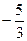degrees per hour. The numberis actually the slope of the line that interpolates between the points (6, 62) and (9, 57). The “exact” linear estimate for 7 p.m. would therefore be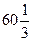degrees. Note: Linear interpolation is valid only for situations where the change is approximately linear. Extrapolation is another way of estimating a y-value on a graph. If you want to estimate the y-value corresponding to a certain x-value that lies outside the domain of data that you have, you can use the trend line already established and “continue to the trend” to make your estimate. For example, if the temperature at 6 p.m. is 62 degrees, and the temperature at 9 p.m. is 57 degrees, what will the temperature be at midnight? If the trend continues (i.e., dropping 5 degrees per every 3 hours), the answer is 52 degrees. Note: Linear extrapolation is valid only for situations where the change is approximately linear and you can reasonably expect that a trend will continue. If the trend cannot be relied upon, or if the x value you are using makes no sense in the context of the problem, don’t extrapolate! Here is an example of extrapolation that makes no sense. When Mr. Hansen was 10 years old, he weighed about 90 pounds. When he was 50 years old, he weighed about 180 pounds. If the trend continues, what will Mr. Hansen weigh at age 90? The “answer” by linear extrapolation is 270 pounds. Clearly, this makes no sense, since Mr. Hansen may not live to age 90, and it’s especially unlikely to happen if he weighs 270 pounds. The “answer” by geometric extrapolation (i.e., doubling weight every 40 years) is even more ridiculous: 360 pounds! A scatter plot (also called scatterplot) is a graph of x-values versus y-values. You place a dot for each ordered pair that associates an x-value with a y-value. For example, a scatterplot of the temperature data given above would require you to plot points at (6, 62) and (9, 57). That’s all it is. A scatterplot allows us to understand the trend (if any) in an immediate, visual way. A scatterplot is much better than a data table for most purposes. The line of best fit is a line that minimizes some of the errors that inevitably occur when we use a straight line in trying to model bumpy, real-world data. You can estimate the line of best fit by sketching it on a scatterplot, but a better way is to enter the x-values into list L1 on your calculator (keystrokes: STAT EDIT to display L1, up and down arrow to enter values into cells) and to enter the y-values into list L2 (same basic technique). Then, you must press 2nd QUIT to return to the main calculator screen. Finally, press STAT CALC 8 L1,L2 ENTER. (Note that you must use the comma key to separate L1 and L2. You enter the names L1 and L2 by using the 2nd function of the numbers 1 and 2 on your calculator keypad.) Note: If you have one of the newer calculators that uses fill-in screens for all the complicated operations, such as STAT CALC 8, you should simply press STAT CALC 8 and then tell your calculator that you want to use L1 for your x-values (“Xlist”) and L2 for your y-values (“Ylist”). Leave “FreqList” and “Store RegEQ” blank. Highlight the word “Calculate” and press ENTER to calculate the line of best fit. Your calculator tells you the line of best fit in a cryptic way: a indicates the y-intercept, and b indicates the slope. So, you have a line, y = a + bx, which you can graph on your calculator as well as on your paper. 2. After you have read the handout and the explanatory paragraphs above, try to complete the handout. You will definitely get stuck. This is normal. Do the best you can, and we will complete the handout in class. Please write a list of specific questions in the margins of your handout or on the reverse side. 3. Continue practicing for the Excelcise. We will conduct our first timed trials during class on Friday. Some people may even pass on the first round! M 4/25/16 No school (Phi Beta Kappa Day). T 4/26/16 C No class. W 4/27/16 D HW due: 1. Continue practicing for the Excelcise. 2. Correct your scatterplot handout (the one you did for last Friday’s class) to 100% perfection by using this solution key. For full credit, please follow these guidelines: (a) Use a different color of ink for your corrections. (b) Write kind but detailed notes to yourself in the margins, addressing yourself by name. BAD EXAMPLE: “Fred, don’t be such a knucklehead!” SLIGHTLY BETTER EXAMPLE: “Fred, don’t be such a knucklehead! Slope is always the value that is being multiplied by x.” GOOD EXAMPLE: “Fred, remember that in the a + bx function that STAT CALC 8 produces, a is the intercept and b is the slope. The slope is always the value that is being multiplied by x.” Th 4/28/16 E HW due: 1. Continue practicing for the Excelcise. A timed trial will be held in class. 2. Complete this worksheet. In #4, you may omit the box plot if you wish. You can answer everything else, however. Feel free to use your calculator or Excel to compute the median, the quartiles, the standard deviation, and so on. F 4/29/16 F HW due (Block 2 only): 1. Read §9.1 (pp. 876-888). Reading notes are required, as always. 2. If you have not already done so, finish the descriptive statistics worksheet that was previously assigned. In particular, make sure in #4 to draw the box plot requested and to compute the interquartile range (IQR). IQR is defined to be Q3 – Q1, i.e., the value at the 75th percentile minus the value at the 25th percentile. In #5b and #6a, note that range (just regular range, not interquartile range) has a different definition in statistics. The definition is max – min, i.e., the largest value in the data set minus the smallest value. You should compute answers for #5b and #6a if you have not already done so. Also note that the definition of the word range in statistics is different from the definition you learned last fall. In statistics, range is a number . . . not an interval or a set of values, the way we would use the word range when talking about the domain and range of a function. You are encouraged to use your calculator to assist you in sketching the box plot. (Use your calculator to display the box plot, and then transcribe a reasonable copy to your HW paper.) Your calculator automatically scales the box plot correctly if you use 2nd STATPLOT and ZOOM 9. 3. Complete this worksheet on lines of best fit and their slopes. M 5/2/16 G HW due (Block 3 only): See calendar entry for 4/29. T 5/3/16 A HW due: Review problems. Do as many of the problems listed below as you can. Keep a time log. For any that you do not finish, be sure to work them before the test on Wednesday as part of your study and preparation. 1. Chapter 8 review (pp. 869-870): #1-19 odd, 23, 27, 41, 45, 51, 53, 59, 60, 67, 68. Probability review: 2. Three fair coins are flipped. Compute (a) the probability of three heads (b) the probability of at least one head (c) the probability of obtaining head, head, tail (in that exact order). 3. Five cards are drawn from a well-shuffled deck (without replacement). Compute (a) the probability that all of them are face cards (J, Q, or K) (b) the probability of at least one face card (c) the probability of at least one ace. 4. Assume that the probability of mumps is 0.1, the probability of chicken pox is 0.2, and the probability of both diseases is 0.05. (a) Are the events of mumps and chicken pox independent? How can you tell? (b) Compute the probability of having neither disease. Make a Venn diagram to help you. (c) Compute the probability of having at least one of the two diseases. (d) Give the correct notation (using union or intersection symbols) for the probability requested in (c). Statistics review: 5. Bamboo grows incredibly fast. Some species can grow several feet per day. Here is a dataset of x-values (elapsed time in days) versus y-values (height of bamboo stalk in inches).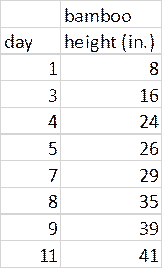(a) Compute the mean, mode, median, and standard deviation of the bamboo height measurements. Use the following notation to label your answers: sample mean =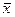= ____________ mode = ____________ median = Q2 = ____________ sample s.d. = s = ____________ (b) Compute the 5-number summary of the height measurements, and make a modified boxplot. (c) Find the line of best fit (linear regression line), and give its equation. (d) Use the line of best fit to estimate the bamboo height at 6 days and at 15 days. (e) Which estimate in part (d) do you trust more, and why? Use the terms interpolation and extrapolation in your answer. (f) Make a scatterplot with the line of best fit overlaid. Be sure to label your axes appropriately. (g) State the slope of the line of best fit. What are its units? (h) Interpret your answer to part (g) in context. (Note: This is an essential PSAT/SAT skill.) In class: Review. Here is a solution key to many of the review problems. W 5/4/16 B Test (100 pts.) on 3 of the following 4 topic areas: Trig, Vectors, Probability, and Statistics. The fourth topic area will be given to you as a take-home worksheet. To help you in your study: solution key to many of the review problems. Important ROOM CHANGE announcement: Because our regular classroom will be unavailable today (on account of a Japanese AP exam), your test will be held in a different location. The Block 2 test will be held in MH-314. The Block 3 test will be held in LJ-302 (the room at the top of the stairway found at the extreme end of the Red Hallway of the Lane-Johnston Building). Th 5/5/16 C No class. F 5/6/16 D HW due: Your take-home portion of Wednesday’s test was e-mailed to you on Thursday morning and is due at the start of class today. Click here for instructions. M 5/9/16 E HW due: 1. Read §9.2 (pp. 893-900). Reading notes are required, as always. 2. Write #5 and #31 on p. 901. Do both problems by hand, using the adding-and-subtracting techniques illustrated in class last Friday. 3. Use your calculator (see instructions in margins of pp. 894-897, or watch this 4-minute video) to solve #8 and #12. No need to show any work; simply write your answer as a set of ordered triples, using the format {(##, ##, ##)}. For example, if your solution is x = 2, y = –14, and z = 3, you would write      {(2, –14, 3)} as your answer, since that is a set of ordered triples. T 5/10/16 F HW due (Block 2 only): 1. Read §9.5 (pp. 928-933). Reading notes are required, as always. 2. Write §9.2 (pp. 901-904) #8-32 eoe, 37, 38, 47, 48, 52. Use your calculator’s rref feature to do all the heavy lifting, but do show all your setup work, especially the process of obtaining the linear system for #32, 37, 38, and 52. “I don’t know” and placeholders will not be accepted as adequate for #32, 37, 38, and 52. At a minimum, you must send an e-mail describing the nature of your confusion, speak with a classmate, or consult some of the example problems earlier in the section. W 5/11/16 G Field Trip. Depart STA (bus on service road near Martin Gym) shortly after 8:00 a.m. Regular school dress is required. We will be back by 1:00 p.m., in time for lunch and your regular Block 6 class period. Any Block 3 student who does not go on this field trip should report to MH-211 (the STAySmart Center, Mr. Findler’s room) at the usual time for roll call and an assignment that you will work on in the library. Your assignment is to write the inequalities that produced each of these graphs, and then begin working on the rest of tomorrow’s assignment. HW due (will be collected tomorrow from Block 3): See calendar entry for 5/10. Th 5/12/16 A HW due: 1. Write the inequalities that produced each of these graphs, 2. Write problems 1, 2, 3, and 4 from this worksheet. Note that #1 and #3 have already been largely done for you; try to do the problems on your own, but if you get stuck, you can refer to the hints at the end for help. A writeup is required for all 4 problems. Important: Your writeup must include a list (or table) of corner points and the objective function value for each corner point; those are not provided in the hints at the end of the worksheet. Inequality sketches should be neat (no scribbling) but are not required to be on graph paper. The purpose of the diagrams is to help you find the corner points so that you can check each corner point in the objective function to find the maximum (or minimum). You are allowed to use desmos.com/calculator to double-check your graphs. Remember, though, that on the final exam, you will be required to do all work either by hand or with your graphing calculator. 3. Finally, remember that if you are in Block 3, there was an additional assignment that was due yesterday. (See the calendar entry for 5/10.) F 5/13/16 B HW due: 1. Finish the inequalities worksheet and the  linear programming worksheet that were due yesterday. Make sure that for each linear programming problem, you include a table similar to the following example for #1: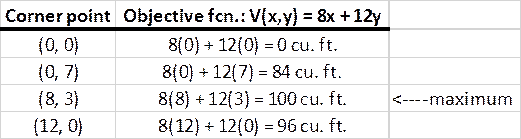As shown in the example above, please use an arrow to mark the maximum (or minimum, depending on the problem). 2. Answer this question: Why does it suffice to check only the corner points of the feasible region? After all, the feasible region in problem #1 (as in most linear programming problems) includes infinitely many points. Why are we able to get away with checking only the corner points? A few complete sentences are expected. 3. Also answer this question: What if the corner point having maximum value of the volume objective function in #1 had had coordinates of (8, 2.7) instead of (8, 3)? Would you still accept (8, 2.7) as the solution for (x, y), or would you require your solution to have both x and y be integers? Note: Optimization problems in which one or more of the variables are required to be integers are called integer programming problems. If the objectives and constraints are all linear, but one or more of the variables are required to be integers, the problem is called an integer linear programming or ILP problem. We will not study ILP or integer programming in general, because those topics are too advanced for Algebra II. However, as you might imagine, ILP and integer programming have many real-world applications. (Think: airline scheduling, concert tour scheduling, contingency staffing, emergency response planning, traffic light timing, urban design, warfighting, political science, marketing, etc., etc.) M 5/16/16 C Field Trip (alternate date). Depart STA (bus on service road near Martin Gym) at 8:00 a.m. Regular school dress is required. We will be back by 1:00 p.m., in time for lunch and your regular Block 1 class. T 5/17/16 D Quiz (40 pts.) on Inequalities, Linear Systems, and Linear Programming. You will use your calculator for most of the questions. However, there will be a few questions in which you are required to do something without your calculator. For example, you may be required to solve a system of 2 equations in 2 unknowns by hand, or you may be required to graph the solution set of a system of inequalities. All linear programming questions will be done with calculator. W 5/18/16 E No additional HW due today. In class: Review, followed by the Scale Model of the Solar System! Th 5/19/16 F HW due (Block 2 only): 1. Write p. 943 #1-25 all. Calculator is permitted for questions 12, 13, 14, 21, 22, and 24 ONLY. All other questions are to be done without calculator (except possibly to check your answers). 2. Note: Some of these problems have already been done in class. That does not matter; write them up neatly on your HW paper. At least you should be able to get those ones correct! 3. For a few of the questions, which are from the precalculus curriculum, exercise your metaknowledge and simply write, “PRECAL.” Do not solve these problems. 4. Keep a time log. Your homework will be graded, not merely spot-checked. In class: Review. F 5/20/16 G End of Q4. All bonus points (crossword puzzles, etc.) must be submitted by 3:00 p.m. today. HW due (Block 3 only): See calendar entry for 5/19. In class: Review. T 5/24/16 Final Exam, 2:00–4:00 p.m., Trapier Theater. Mr. Hansen’s office hours for exam week are Monday 10:00-2:00, Tuesday 10:00-1:45, and Thursday 10:00-2:00. If you can’t finish your Excelcise and/or Must-Pass Quiz during those times, you need to contact Mr. Hansen ASAP! Resources To assist you, here are some resources you may find helpful:   ·         ·         ·         ·         ·         ·         ·         ·         ·         Study Guide In addition to the cumulative sample test on p. 943, you will want to make sure you have covered the following areas/topics in your exam review:   ·         Probability (including permutations, combinations, counting rules, Venn diagrams, multiplication rule, general union rule, general intersection rule, and conditional probability) ·         Statistics (including mean, mode, median, standard deviation, scatterplots, boxplots, and interpretation of the slope of the line of best fit) ·         Linear programming (including definition of variables/“Let” statement, plotting of the feasible region, and evaluation of the objective function at each corner point) ·         Rules of logarithms (including LPSL, “house rule,” and LQDL rules: log ab = log a + log b, log xa = a log x, and log of a quotient equals difference of logs) ·         Solution of exponential and logarithmic equations ·         Solution and application of trigonometric equations ·         Conversion between radian and degree measure (for example,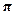radians = 180°) ·         Plotting of trigonometric functions (for example, why does y = 3 sin 2x have a period of only, when a typical sinusoid has a period of 2?) ·         Hero’s Formula, law of sines, law of cosines, area of triangle by trigonometry (finding altitude by using a sine function as multiplier) ·         Basic knowledge from semester 1 (including rules of exponents, simplification of expressions, quadratic formula, factoring, inverse functions, and domain and range of functions) F 5/27/16 Update! There will be additional office hours on Friday, May 27, from 7:30 a.m. to 12:30 p.m. The following people need to be sure to stop by during that window in order to pass the Excelcise: Collin Drew Joel Julian Michael

-- New world record for paper folding! Pomona, CA, teenager achieves a seemingly impossible 12 folds!
-- Lots and lots of
online IQ tests
--
Fallacious proof that pi equals 5
-- An extension of the concept of
freshman cancellation
--
National Cryptologic Museum, brought to you by the National Security Agency
-- The famous three houses/three utilities puzzle
-- The amazing nine-point circle
-- Abusive tax shelters: Maybe you will use your education to devise clever schemes like this, or better yet, to try to eliminate them
--

-- Midterm exam study guide and practice exam
-- Angle-arc puzzles written by the Class of 2006 (in 2003)
-- More angle-arc puzzles written by the Class of 2007 (in 2004)
-- Brain teasers written by the Class of 2008 (in 2004-05)
-- Timed math quizzes, courtesy of Mr. Errett
--
Quizzes, quizzes, and more quizzes for geometry and other math subjects (don’t let the title ‘Math for Morons’ discourage you—that’s really a moronic name for the site)
-- Proficiency test for rising 9th graders from the great state of Illinois
-- B.J. Pinchbeck’s
Homework Helper for all subjects